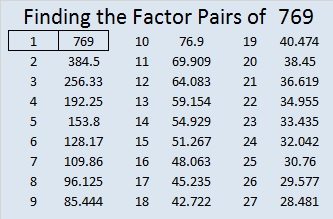# 769 and Level 2

• 769 is a prime number.
• Prime factorization: 769 is prime.
• The exponent of prime number 769 is 1. Adding 1 to that exponent we get (1 + 1) = 2. Therefore 769 has exactly 2 factors.
• Factors of 769: 1, 769
• Factor pairs: 769 = 1 x 769
• 769 has no square factors that allow its square root to be simplified. √769 ≈ 27.730849.How do we know that 769 is a prime number? If 769 were not a prime number, then it would be divisible by at least one prime number less than or equal to √769 ≈ 27.7. Since 769 cannot be divided evenly by 2, 3, 5, 7, 11, 13, 17, 19, or 23, we know that 769 is a prime number.

Here is today’s puzzle:Print the puzzles or type the solution on this excel file: 12 Factors 2016-02-25

————————————-

Here’s more about prime number 769:

25² + 12² = 769, and 769 is the hypotenuse of primitive Pythagorean triple 481-600-769 which was calculated from 25² – 12², 2(25)(12), 25² + 12².

Thus 481² + 600² = 769².

769 is also the sum of three squares five different ways.

• 27² + 6² + 2² = 769
• 24² + 12² + 7² = 769
• 21² + 18² + 2² = 769
• 20² + 15² + 12² = 769
• 18² + 18² + 11² = 769

769 is palindrome 181 in BASE 24; note that 1(24²) + 8(24) + 1(1) = 769.

Here’s another way we know that 769 is a prime number: Since  its last two digits divided by 4 leave a remainder of 1, and 25² + 12² = 769 with 25 and 12 having no common prime factors, 769 will be prime unless it is divisible by a prime number Pythagorean hypotenuse less than or equal to √769 ≈ 27.7. Since 769 is not divisible by 5, 13, or 17, we know that 769 is a prime number.

————————————-This site uses Akismet to reduce spam. Learn how your comment data is processed.